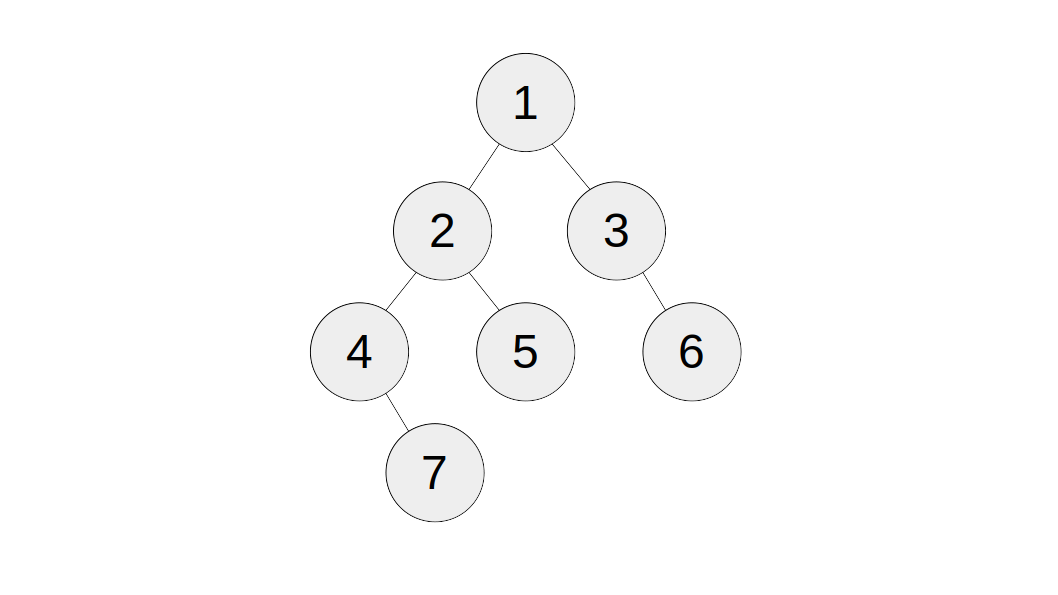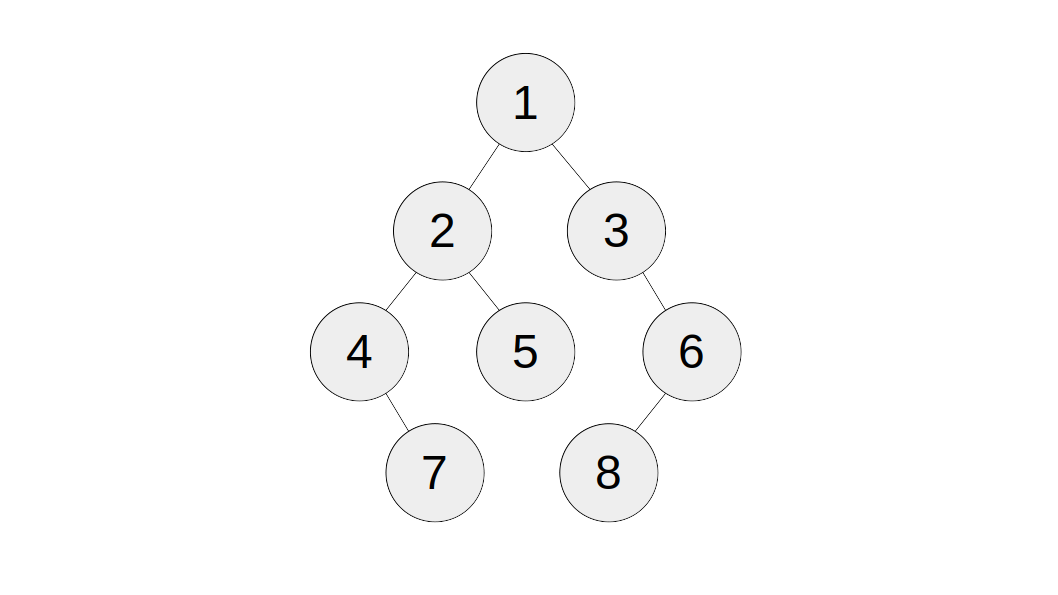# Balanced Binary Tree

A balanced binary tree is defined as a tree such that either it is an empty tree, or all its subtree are balanced and has a height difference of at most 1.

In that case, given a binary tree, determine if it's balanced.

#### Parameter

• `tree`: A binary tree.

#### Result

• A boolean representing whether the tree given is balanced.

### Examples

#### Example 1

Input:Output: `true`

Explanation: By definition, this is a balanced binary tree.

#### Example 2

Input:Output: `false`

Explanation: The subtrees of the node labelled `3` has a height difference of `2`, so it is not balanced.